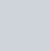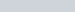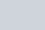• 7
•
• 2
•
•
•
•
•
9
Shares

# INTERNAL RESISTANCE AND ELECTROMOTIVE FORCE

### INTRODUCTION

To complete a circuit, we need a cell or a battery, a wire, a switch, and a load (load is optional, it is your choice that you want to use or not). When we connect these components it forms a closed complete circuit. As the circuit is completed, the current starts flowing from the positive terminal (cathode) to the negative terminal (anode).

We know that every wire or conductor has the property to resist the flow of electric current when it tries to flow through them. We also know that due to this resistance wire becomes hot or sometimes it gets melts.

But did you know? The battery or cell which we use in the circuit also becomes hot if it is used for a long duration. For example, you must have seen that when you use excessively your smartphone then its back area becomes hot and it becomes hotter when you use it during charging. Did you know why it so happened?Did you know? When you purchase a new cell or battery then the potential difference between their terminals is maximum. For example: Assume you purchase a brand new AA cell then its potential difference across their terminals is 1.5V but as you put it into the circuit then its potential difference not the same as it is indicated on it. It is always less than the mark value of potential difference. Did you know? Why it so?

In this article, we are going to discuss internal resistance and electromotive force in detail. So stay tuned with us till the end.

## ELECTROMOTIVE FORCE

Electromotive force: the force which moves the electrons. Wait, this is not the real definition of these terms. It is what the term says.

Let’s ask a question, why did electrons move inside any conductor? You may say because we supply electricity from cell or battery. But what did this electricity do in the circuit? When we supply electricity, it means we are creating potential differences.

This potential difference creates an electric field inside the conductor from cathode to anode. And the electrons flow from the negative terminal to positive terminals i.e from anode to cathode in opposition to the electric field. This motion of the electrons creates an electric current in the circuit.

### ELECTROMOTIVE FORCE: DEFINITION

In any cell or battery, there are two types of potential difference, one is when, when the electric current is flowing through the circuit. And second is when, when there is no electric current flowing through the circuit i.e. when there is no electric current drawn from the cell or battery.

When any battery or cell is in use then the voltage across their terminals is taken as potential difference. But when the battery or cell is not in any use then the voltage across their terminals is called electromotive force.

Electromotive force is not a force but it is a special kind of potential difference measured then when batteries are not in use. We can also define this in the term of work done.

We know that potential difference is work done per unit positive charge, which means the potential difference is the required work done to move a unit positive charge from one terminal to another terminal of the cell or battery. This movement of charge particle only in the circuit not in the cell or battery.

Electromotive force is also a work done per unit charge but in this, charged particle travels through the whole circuit including the cell or battery. So it can be defined as the work done required to move the unit positive charge through the whole circuit including the cell is called electromotive force.

Unit of electromotive force is same as potential difference i.e joule/coulomb or volts. If one joule of work done is needed to move the one coulomb of electric charge through the circuit including the cell then we called it one volt of EMF.

It is denoted by the capital letter E or sometimes it is denoted as the Greek letter epsilon ε.

## INTERNAL RESISTANCE

Remind that we have talked above that when we use smartphones excessively for hours then the back of the phone becomes hotter. This is not only for smartphones every electrical source that continuously supplies power to the loads, then becomes hot. So what is the reason behind it? Let’s find out.We know that wire or any electrical conductor has the property to resist the flow of electric current through them. But what only wire or conductor has the property to resist electrical circuit?

We know that current exit from the positive terminal and entered through the negative terminal (magnitude remains the same) but what about the middle path of the cell or battery. Does it not resist the flow of electric current? Yes, it does. If it does not resist the flow of current then why did it get hot? Their becoming hot tells that they also resist the flow of electric current.

So the resistance offered by the electrolyte or inner materials of the cell is called internal resistance. This internal resistance depends upon several factors, some of which are.

• It depends on the separation distance between the plates or terminals.
• It depends on the surface area of the electrode if it is dipped into any electrolytic solutions. But if it is a dry cell then it depends on the cross-sectional area of the carbon rod.
• It depends on the nature, temperature, and concentration of the electrolytes and it increases if the concentration increases.

The internal resistance of the cell or battery is measured in ohms. It is denoted by the small letter r.

## RELATION BETWEEN EMF, POTENTIAL DIFFERENCE, AND INTERNAL RESISTANCE

EMF is the maximum potential difference across the terminals of the cell. But when it is in use, this EMF breaks into several potential drops, such that if all the potential drop is summed then it becomes equal to the EMF of the cell.

Consider a circuit having resistance R, a cell having EMF E and internal resistance r and potential difference of the cell is V, then Mathematically

(1)The current in the circuit can be also given as-

(2)Equating equ(1) and (2), we get-This is the formula for the internal resistance of the cell.

## DIFFERENCE BETWEEN EMF AND POTENTIAL DIFFERENCE

We have talked about EMF and potential differences and we have seen that there is no much distinction between them. EMF is the potential difference when the circuit is open and voltage is the potential difference when the circuit is closed.

In the table, we are listing some distinctions between them.

 S.No Electromotive force Potential difference 1 It is the amount of work done required to move a unit positive charge through the whole circuit including the cell. It is the amount of work done required to move a unit positive charge through the circuit excluding the cell. 2 It is the maximum voltage of the cell when the current is not drawn from the cell. It is the voltage of the cell when the current is drawing from the cell. 3 It is independent of the resistance of the circuit. It depends on the resistance of the circuit. 4 It depends on the nature of the electrodes and the nature of the electrolyte. It depends on the value of the resistance and the value of the current flowing through them. 5 Its SI unit is Volts. Its SI unit is also Volts. 6 It is denoted as capital E or sometimes it is denoted by a greek letter epsilonIt is denoted by the capital letter V. 7